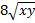To multiply radical expressions (square roots)... 1) Multiply the numbers/variables outside the radicand (square root) 2) Multiply the numbers/variables inside the radicand (square root) 3) Simplify if needed Examples: 1)On the outside, 5.3=15 and on the inside, 2.7=14. The radical cannot be simplified further. 2)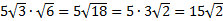On the outside, we only have a 5, so that stays the same (or you can think of it as 5.1). On the inside, 3(6)=18.can be simplified toSince the 5 and 3 are both on the outside, we finish by multiplying them together. 3)When variables are involved, we use the same process. Since there were two x's inside, we could take that outside the square root. Practice: Multiply the radicals. 1)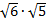2)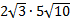3)4)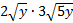5)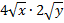Answers: 1)2)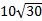3)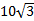4)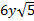5)